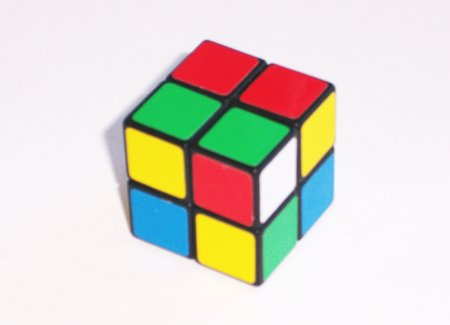## news from Rubik’s cubeHomework: how many permutations has the above pictured cube?

A regular Rubik’s cube has 43,252,003,274,489,856,000 permutations (different positions) and it is still unknown how many moves are needed to solve the cube, that is to find a shortest path from a given permutation to the trivial one. If you count turning a face as a move it is known that there are permutations that need 20 moves. This is a lower bound meaning that one knows that there are permutaions that need at least 20 moves, but there might even be permutations that need more. Last year the upper bound by Silviu Radu was 27 moves but Dan Kunkle and Gene Cooperman now showed that it can be done faster: With massive computer aid they showed that 26 face moves are enough.
So the situation is: there are permutations that need 20 or more moves and any permutation can be solved with at most 26 moves. The gap of 6 between these two numbers gives us the following bounds: We can expect at least one more paper and at most 6 more papers that reduce the gap.

The above pictured pocket cube has only 3,674,160 permutations and optimal solutions for all of them can be generated by a brute force algorithm.
this is an update to The eighties recycle themselves and rubiks robots.

The below box is for leaving comments. Interesting comments in german, french and russian will eventually be translated into english. If you write a comment you consent to our data protection practices as specified here. If your comment text is not too rude and if your URL is not clearly SPAM then both will be published after moderation. Your email adress will not be published. Moderation is done by hand and might take up to a couple of days.
you can use LaTeX in your math comments, by using the [latex] shortcode:
[latex] E = m c^2 [/latex]